We’d like to understand how you use our websites in order to improve them. Register your interest.

# Experimental study on tsunami attenuation by mangrove forest

## Abstract

Laboratory experiments on the effectiveness of mangroves to reduce tsunami energy were performed. A complex tree structure of Rhizophora sp. was parameterized using the stiff structure assumption (root system and trunk) for different submerged root volume ratios and frontal tree areas. The hydraulic resistance of the prototype and the parameterized models under steady flow conditions was compared and the most appropriate parameterized model in terms of both equivalent flow resistance and practical feasibility was selected for further investigation. The damping performance of the mangrove forest was determined from laboratory tests performed synchronously in a twin-wave flumes (with and without the forest model in 1 and 2 m-wide wave flumes, respectively) for varying incident height of solitary wave, water depth and forest width. The role of the different types of wave evolution modes on wave damping is discussed based on the measurements of the forces exerted on the single tree models along the entire forest width. A new approach for the wave transmission coefficient, which is based on the ratio of the forces exerted on the trees placed in the last and first forest row, is proposed. In the paper, the most important results of the tree parameterization procedure and the wave flume experiments are discussed.

## 1. Introduction

The capability of coastal forests to reduce the impacts of extreme events such as tsunamis and storm surges has been reasonably considered by engineers and scientists as one of the risk mitigation measure alternatives. In some degree, typical coastal forest vegetation such as mangroves or coastal pines are generally sufficient to withstand extreme winds or storms. However, the capability of the forests to withstand extremely high tsunami has controversially been discussed due to the fact that so far, there are no definite conclusions, particularly on the role of the coastal forests as an effective natural tsunami barrier. Several reports and surveys (field observations, experienced damage, and satellite images) have apparently shown that coastal forests may play an important role as a natural protection against tsunami (Dahdouh-Guebas et al., 2005). These findings are also supported by semi-analytical and empirical approaches based on series of experiments using either physical or numerical models (Istiyanto et al., 2003; Imai and Matsutomi, 2005; Yanagisawa et al., 2009).

On the other hand, many unknown aspects still remain unknown to draw any conclusions on the effectiveness of coastal forest vegetation as a protective green-shield. Field evidence (damage surveys) also showed that coastal forests did not always effectively protect coastal areas from destruction by tsunami (Chatenoux and Peduzzi, 2005). Some tree species did survive tsunami attack, however the villages behind them experienced significant damages (Tanaka et al., 2007). Even in some areas with dense and healthy mangroves (e.g. Ule-lhe, Banda Aceh, Indonesia), the forest did not provide any protection against the 2004 tsunami. Instead, the trees were destroyed, uprooted, and carried kilometres inland, creating more lethal tsunami debris (EJF, 2006). Moreover, tsunami onshore propagation, the effect of complex bathymetry and topography, and relevant vegetation characteristics are some of the poorly understood aspects related to the damping performance of coastal forests.

One of the important aspects associated with the attenuation performance of coastal forest against tsunami is the hydraulic resistance of the vegetation, which determines the overall tsunami attenuation (transmission, reflection and dissipation) by coastal forests. Efforts to derive the hydraulic resistance in terms of drag, inertia or Manning roughness coefficients have been made mostly via series of laboratory experiments (Harada and Imamura, 2000). To provide reliable values of the hydraulic resistance from laboratory tests, the complex tree structure has to be simplified first through a parameterization process, based on the assumption that the hydraulic resistance of the prototype tree and the parameterized tree model is identical. However, the methodologies used to parameterize the vegetations were often not physically-based and contradictory to each other (Husrin and Oumeraci, 2009). To date, there is no general consensus for a proper selection of the hydraulic resistance for typical coastal forest vegetation.

Another aspect that has not been fully understood is the distinction between tsunami attenuation by shore topography and coastal forest. The presence of natural complex bathymetry/topography (e.g. natural reefs or dunes) may significantly contribute to the attenuation of tsunami energy in comparison to that of the coastal forest (Chatenoux and Peduzzi, 2005). Strusińska (2011) has concluded that the dissipation of tsunami-like solitary wave by submerged reefs is influenced not only by wave conditions but also by reef geometry and local water depth conditions, affecting generation of wave breaking. Different shore topography (i.e. different beach slopes) has also been examined in laboratory experiments to determine the attenuation of tsunami by forest models (e.g. Istiyanto et al., 2003; Kongko, 2004; Imai and Matsutomi, 2005). However, the distinction between the energy dissipation due to the forest and the shore topography, including the associated physical processes such as inception of wave breaking, was not clearly shown.

In this paper, a concept for the parameterization of mangrove trees (Rhizophora sp.) with stiff structure assumption (only roots and trunk) and their associated hydraulic resistance coefficients will first be discussed, including some aspects not considered so far in the literature and which have been identified based on the recent laboratory tests. Furthermore, the most first results of the large scale model tests on the damping performance of the forest, consisting of individual parameterized mangrove tree models, will be presented.

## 2. Developed Procedure of Tree Parameterization

Parameterization is a simplification process of a complex 3D structure of coastal forest vegetation to a simpler and more organized model with a similar resistance to flow, i.e. the hydraulic losses of the prototype should be similar to the hydraulic losses of the proposed parameterized model. Husrin and Oumeraci (2009) have identified the most important parameters and aspects for the parameterization of typical coastal forest vegetations; mangroves and coastal pines. Not only geometrical aspects should be considered in the vegetation parameterizations, but also other physical and biological aspects such as tree species, age, stiffness, frontal area, canopy density (characterised by the leaf area index LAI), natural frequencies, and behaviour under seasonal changes.

Considering the physical and morphological aspects of the vegetation, three main parts of a tree are addressed in the parameterization: roots, trunk and canopy. Each structural part of a tree contributes simultaneously in blocking/reducing the flow impact. For tsunami height lower than the canopy, the trunk and roots play a dominant role in the flow reduction; this is particularly the case for the complex root system of mangroves (Rhizophora sp.). However, when tsunami reaches the canopy, all parts of the tree become important. The canopy with much higher density may result in higher wave damping, provided the trunk is strong enough (not broken) and the roots are well anchored in the soil (not uprooted). These characteristics (the canopy density, the trunk strength, and root parameters) depend on the vegetation species, age, and environmental aspects. At younger age, any species are vulnerable to damage (breaking or uprooting), while at a mature stage, trees are structurally more stable but still vulnerable to breakage. Therefore, two assumptions have been made for the parameterization process: (i) stiff structure assumption, in which trunk and roots of the tree are considered, (ii) flexible structure assumption which is applicable to all tree components (i.e. trunk, roots, and canopy). In this paper, the parameterization of a mangrove tree (Rhizophora sp.) according to the stiff structure assumption will be discussed in more details, including the derivation of the hydraulic resistance based on results obtained from laboratory experiments. This assumption results from the fact that most of the bottom parts of mature mangroves (Rhizophora sp.) remain intake after being hit by large tsunami (Yanagisawa et al., 2009). Furthermore, for the case where tsunami does not reach the canopy, the roots and the trunk behave relatively stiff.

Three mangrove models of complex roots with different density, analogue to the prototype, were constructed at a scale of 1:20. These are called hereafter ‘real tree models’ or models A1, B1, and C1 (see Fig. 1). The dimensions of the roots refer to the work of Istiyanto et al. (2003) and are based on the field measurements of the mangrove forest in Jakarta Bay, Indonesia by the first author. The height of a mature Rhizophora sp. is typically 15 m from the bottom and the height of the root system for a 15 m high tree is 2.50 m. The submerged volume ratio of these models is within the range reported by Mazda et al. (1997).

For each real model, three parameterized models made of a group of cylinders with different diameters were constructed. The different diameters indicate the influence of different frontal area A f , submerged root volume ratio V m /V, and cylinder dimensions on the hydraulic performance of the models. The frontal area A f (or blockage area) is defined as the area perpendicular to the flow direction of the submerged tree model. Hence, the frontal area is determined by taking the picture of the intended side of the model to be subjected by the flow (Fig. 1). The submerged root volume ratio is defined as a ratio of the submerged root volume (V m ) related to the water control volume (V) (Mazda et al., 1997). Both the frontal area and the submerged volume ratio vary as the water level changes. Figure 1 also shows real mangrove models and their counterpart parameterized models with the nomenclature used.

A flume with steady flow was used to measure the hydraulic properties of the models. The models and the measuring devices (current meters, surface elevation gauges, and a force transducer) were installed in the middle of the flume (see Fig. 2). With a scale of 1:20, Froude’s similitude law was applied to obtain the required flow velocities (discharges) for the whole series of the experiments. Maximum measured tsunami onshore velocities range from 2–5 m/s (Fritz et al., 2006) so that the required current velocities in the model should be at least within the range of 0.4–1.0 m/s. Flow velocities were measured using Acoustic Doppler Velocimeters (ADV) in front of and behind the model. Surface elevation was measured at three locations: in front of, at and behind the mangrove model. Hydraulic forces were measured by means of a force transducer mounted to the bottom of the tree model.

All models were tested in the flume according to the experimental set-up shown in Fig. 2. Different flow velocities ranging from u = 0.2–1.4 m/s (or relative flow discharges q = 0.2–1.4 m3/s/m2) for four water depths (h = 0.05, 0.10, 0.125 and 0.15 m) were employed in the testing programme to investigate the effect of varying frontal area A f and submerged root volume ratio V m /V on the measured hydraulic forces. The flow velocity is measured at the level of at least 0.4 h above the platform which can be considered as the depth-averaged flow velocity. Each model was subjected to a combination of different current velocities and water depths in 17 tests. Therefore, the total number of tests is 204 (17 tests × 12 mangrove models).

The measured hydraulic forces are plotted in Fig. 3 for different root densities. The effect of the root density is clearly visible—the force increases as the root density increases. The measured force increases by about 65% from 8.5 N for the lowest root density to 14 N for the highest root density. It is also clear that the size of the cylinder diameter, used to represent the mangrove root system, influences significantly the measured forces—as the root density increases, the effect of the cylinder diameter decreases. The cylinder diameter D c = 0.5 cm, which is close to the averaged root diameter of the real model, shows consistent results for all considered root densities.

Comparison of the measured forces for the real and the parameterized models is necessary to identify which parameterized model is most appropriate to represent its actual counterpart (i.e. the real model). Therefore, the deviation from the real model represents a very important parameter for the selection of the appropriate parameterized model. The deviation of the measured forces on the parameterized models from that on the real models is calculated as follows: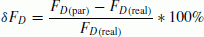((1))

where δF D is the deviation of the measured force, FD(par) the measured force exerted on the parameterized model and FD(real) the measured force exerted on the real model.

In terms of the force measurements, the parameterized models with cylinder diameter 0.5 cm provide a better representation of the real model with a deviation about –13% (see Table 1). Moreover, the force measurements showed much more reliable and conclusive results. The measured forces for both real and parameterized models are comparable and consistent with the physical characteristics of the respective models (i.e. density and frontal area). The flowinduced force is a function of both flow regime and the characteristics of the body in the flow. Therefore, considering the discussion above, the parameterized model with cylinder diameter D c = 0.5 cm is the most representative one.

Large force deviations, attributed to the parameterized models with larger cylinder diameters (D c = 1.0 and D c = 1.5 cm) may result from the different cylinder geometry used, which affect the magnitude of the flow-induced forces. The models with D c = 1.0 cm are subjected to larger forces (averaged deviation of δF D = 42%) in comparison to the models with the largest cylinder diameter (D c = 1.5 cm) and with clearly smaller forces measured (averaged deviation, δF D = −35%), as shown in Table 1. The weaker flow-induced load on these models is caused by larger spaces among individual cylinders, allowing the flow to pass more freely through the models (see Fig. 1).

According to the Morisson equation, the total hydraulic force of a body subject to flow consists of drag and inertia forces. In the parameterization study, the inertia component is not considered because the flow is quasi-steady (i.e. the changing rate of flow velocity du/dt during the experiments is negligibly small). Therefore, the total hydraulic force for the current study can be simplified as follows: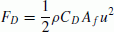((2))

with drag coefficient C D calculated as: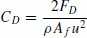((3))

where A f is the frontal area of the mangrove model, F D the measured drag force, u the current velocity and ρ the water density.

To validate the reliability of the parameterized models, the hydraulic resistance in terms of drag coefficient C D is investigated further according to Eq. (3). Mazda et al. (1997) proposed a relationship between C D and Reynolds number R e , in which the Reynolds number has been redefined for the mangrove root system. Therefore, the characteristic length scale in the original definition of the Reynolds number should be replaced by an effective length L e , which can be interpreted as a measure of porosity expressed in a length scale. Larger L e values mean smaller root density and smaller L e values mean larger root density. The effective length of a mangrove root system is defined according to the concept of submerged root volume ratio V m /V and frontal area A f :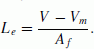((4))

Therefore, the Reynolds number becomes: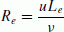((5))

where L e is the effective length, u the averaged flow velocity, V the water control volume, V m the submerged root volume and ν the kinematic viscosity of water (ν = 1.004 × 10−6 m2/s for water temperature of 20°C).

The C D values, plotted against effective length L e in Fig. 4, clearly show the influence of both the submerged root volume ratio and the frontal area. The frontal area which is intrinsically included in the L e obviously influences the C D . As the A f increases, L e decreases and the C D generally increases as well. The F D of Models C are generally larger than Models B and C. The C D increases for smaller L e (higher root density and larger A f ) for all mangrove models and it decreases towards 1.0 as the effective length increases (lower root density and smaller A f ). This means the higher L e values lead to a more sparse root system. Thus, at this condition, the root system may act as a single cylinder because the associated C D is equivalent to the C D for a single cylinder (C D = 1.0 for R e = 102–105). The results shown in Fig. 4 are comparable with other measurements such as those presented by Grant and Nickling (1998). Higher root density (smaller L e ) is associated with higher drag coefficients due to the fact that the flow may skim around the object instead of penetrating through the root system. At some points, the flow interaction within the roots (or a group of cylinders) may experience pressure losses in the wake, thus resulting in higher C D values. In contrast, lower root density (higher L e ) allows the flow to penetrate freely into the root system without loosing much of the momentum and low wake interference.

The recent study is also comparable to the previous experiments dealing with mangrove models and their hydraulic resistance. Harada and Imamura (2000) conducted laboratory experiments using artificial porous media for parameterized mangrove models and proposed a relationship for C D versus V m /V. Though, the physical basis of the relationship is unclear, incomplete and found to be in the lower envelope of the current study (Husrin and Oumeraci, 2010). The obtained hydraulic resistance from the current study also confirms the laboratory works of Struve et al. (2003) for higher C D values and Imai and Matsutomi (2005) for smaller C D values (C D ~ 1.0).

Moreover, a new relationship for the flow resistance in terms of drag coefficient C D can be derived as a function of Reynolds number (see Fig. 5): for the upper envelope: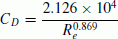((6))

and for the lower envelope: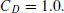((7))

Combining all of the obtained data from the experiments with the real models (models A1, B1 and C1) and the parameterized mangrove models (models A2, B2 and C2), the optimum relationship of C D as a function of the Reynolds number can be derived as follows: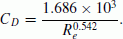((8))

The relationships shown in Eqs. (6)(8) and in Fig. 5 explain thoroughly both physical object properties and flow characteristics such as variation of root density V m /V, frontal area A f , and flow regimes. Therefore, the parameterized model, which has been selected in the current study, is physically based and sufficiently verified under steady flow conditions to be further implemented for a larger scale of model experiments on tsunami attenuation by a mangrove forest under a stiff structure assumption in a wave flume, described in the next section.

## 3. Wave Flume Experiments on Tsunami Attenuation by Mangrove Forest

The effect of a mangrove forest on wave attenuation was examined by performing synchronous experiments in the twin-wave flumes of the Leichtweiss-Institute (LWI) at the Braunschweig University of Technology (Germany), which consists of 1 m- and 2 m-wide parallel flumes, both approximately 90 m long and 1.2 m high. For this purpose, a typical coastal cross-shore profile with and without the mangrove forest model was constructed in the 2 m- and the 1 m-wide flumes, respectively (see Figs. 6(a) and (b)). The sloping part of the cross-shore profile (~1:20), built at a distance of 23.64 m from the wave maker, represents a foreshore slope, which evolves into a horizontal platform of height 0.415 m, containing the parameterized mangrove forest model.

The forest model was arranged in staggered rows of 12 and 13 tree models, as shown in Fig. 6(b). Thus, the forest density was kept constant and only the forest width was varying (B = 0.75, 1.5, 2.25 and 3.0 m as well as B = 0.0 m corresponding to no forest in the 1 m-wide flume). By varying the water depth in front of the foreshore model (h = 0.415, 0.465, 0.515, 0.565 and 0.615 m), different submergence depths of the forest were achieved, covering the entire tree model height (i.e. up to the top of a trunk in case of the stiff tree structure): the tree models were fully emerged for d r = 0.0 m and fully submerged for d r = 0.2 m (d r : water depth over the horizontal part of the beach platform).

However, the lowest water level (d r = 0.0 m) was further excluded from the experimental programme, since waves did not reach the forest front and thus no wave transmission through the forest was observed. The same solitary waves of varying incident nominal wave height H i,nom = 0.04− 0.20 m (with an increment of 0.04 m) were generated synchronously in both wave flumes (Table 2).

The complex wave-forest interaction required diverse measuring techniques and the development of new devices adjusted to the testing conditions (see Fig. 7). More than 10 force transducers recording wave-induced forces on single tree models (FTS) and one force transducer recording forces exerted on the entire forest model (FT) were designed, constructed and calibrated. The measurements of the forces exerted on the tree models were performed through a direct connection of the device to the tree model as shown in Fig. 6(d) (ensuring free movement of the tree in the direction of wave propagation). In order to record the forces exerted on the entire forest model, a steel frame containing the mangrove models, moveable in the direction of wave propagation, was attached to the force transducer, as shown in Fig. 6(e). More than 30 wave gauges were deployed to determine the wave evolution along the wave flumes, while information on the pressure and the velocity fields at the forest was provided by pressure transducers (PT) and Acoustic Doppler Velocimeters (ADV), respectively (see Figs. 6(a) and (c)).

## 4. Classification of Wave Evolution Modes

Two main sources of wave damping have been recognized in the performed tests: (i) due to the wave interaction with the mangrove models, particularly with the root system, (ii) due to wave breaking generated for favorable water depth/incident wave conditions. In order to identify the origin of the wave energy reduction and to properly assess the wave damping performance of the mangrove forest, a classification of the wave evolution modes is introduced for waves propagating in the 2 m- and 1 m-wide flumes (i.e. with and without the forest model, respectively). The criteria employed to characterized the modes of wave behaviour were:

1. (i)

generation of wave breaking,

2. (ii)

location of incipient wave breaking occurring in four regions along the beach profile as shown in Fig. 8 (i.e. in region 1 over the foreshore slope, in region 2 stretched between the end of the foreshore slope and the beginning of the forest model, in region 3 corresponding to the forest width, in region 4 behind the forest model),

3. (iii)

generation of wave fission resulting in a train of solitary waves emerging from a single incident solitary wave (consisting of at least two solitons), caused predominantly by the water depth reduction from h = 0.465–0.615 m in front of the beach profile to d r = 0.05–0.20 m above the horizontal part of the beach model, and also due to the presence of the forest model.

Based on the free surface measurements and video recordings, the following wave evolution modes have been distinguished:

• Non-breaking waves:

• Non-breaking wave disintegrating into solitons (evolution mode “EM1”). No wave breaking is generated as the wave propagates over the entire foreshore model. However, the wave splits into a number of solitons (see Figs. 8(a) and 9). This evolution mode was observed for two smallest incident wave heights: H i,nom = 0.04 m at water depth h = 0.515–0.615 m and H i,nom = 0.08 m at water depth h = 0.615 m.

• Breaking waves:

• Breaking of incident wave over the beach slope (i.e. in region 1) with wave disintegration into solitons (evolution mode “EM2”). The progressive broken wave resembles a turbulent bore (see Figs. 8(b) and 9). Due to the complex shape of the broken wave, recognition of wave fission generation was in some cases not successful and thus a longer travel distance would be required for a full development of the solitons. A promising analysis method for this purpose is the non-linear Fourier Transform proposed by Brühl and Oumeraci (2010). This evolution mode is typical for the smallest water depth h = 0.465 m and relatively high waves H i,nom = 0.12–0.20 m.

• Breaking of incident wave in the region between the end of the beach slope and the beginning of the forest model (i.e. in region 2) with wave disintegration into solitons (evolution mode “EM3”) (Figs. 8(c) and 9). This evolution mode was found to be the mostly often observed pattern in the tests. Depending on the inception point of the wave breaking, two submodes can be further distinguished: (i) generation of wave breaking followed by wave fission, provided breaking event was generated very close to the end of the foreshore slope (evolution mode “EM3a”), (ii) wave disintegration into solitons followed by wave breaking occurring for the tests in which the inception point of breaking was shifted towards the beginning of the forest (evolution mode “EM3b”).

• Breaking of incident wave in the forest model (i.e. in region 3) with wave disintegration into solitons (evolution mode “EM4”). The process of wave scattering into solitons takes place already in front of the forest. As a result of the increase of the height of the leading soliton, which accompanies wave fission, the first wave becomes unstable and breaks in the forest. Further development of the fission process starts once the breaking event is accomplished (see Figs. 8(d) and 9). This evolution mode was observed for forest width B = 1.5–3.0 m, two highest water levels h = 0.565 and 0.615 m and incident wave height range of H i,nom = 0.08–0.16 m.

• Breaking of incident wave behind the forest model (i.e. in region 4) with wave disintegration into solitons (evolution mode “EM5”). Similarly to “EM4”, the incident wave starts to scatter into solitons in front of the forest: The leading soliton becomes unstable due to the wave height amplification associated with the fission, and breaks behind the forest. Further evolution of the solitons can be observed within and behind the forest (Figs. 8(e) and 9). This evolution mode was observed solely for the shortest forest of width B = 0.75 m, for waves of height H i,nom = 0.08 m generated at water depth h = 0.565 m and H i,nom = 0.12 m at h = 0.615 m.

The effect of water depth, incident wave height and forest width on the solitary wave evolution modes observed in the 2 m-wide flume is illustrated in Fig. 9. The change of the two first parameters was found to influence significantly the pattern of wave behaviour, particularly on the transition from the breaking to the non-breaking wave conditions. Generally, smaller waves (H i,nom = 0.04, 0.08 m), generated at the lowest water depth (h = 0.465 m), broke in front of the forest (“EM3”), while higher waves (H i,nom = 0.12–0.20 m) broke earlier, already over the foreshore slope (“EM2”). By increasing the water depth to h = 0.515 m, non-breaking wave conditions (“EM1”) were achieved solely for the smallest wave height (H i,nom = 0.04 m), while waves of height H i,nom = 0.08–0.20 m broke in front of the forest (“EM3”). In this case, waves of height H i,nom = 0.08 m, which were classified as “EM2” for h = 0.465 m, became “EM3”. For higher water level of h = 0.565 m, the same wave behaviour as for h = 0.515 m was observed except for waves of height H i,nom = 0.08 m (breaking behind forest—“EM5” for forest width of B = 0.75 m and breaking in the forest—“EM4” for the other forest widths). In the case of the highest water depth h = 0.615 m, non-breaking wave conditions occurred for two smallest wave heights (H i,nom = 0.04, 0.08 m). In comparison to the previous water depth, waves of height H i,nom = 0.12 m broke behind the forest of width B = 0.75 m and in the forest of width B = 1.5–3.0 m. Except of waves of height H i,nom = 0.08, breaking in the forest of width B = 1.5 and 2.25 m, the evolution modes of the two highest wave heights remained unchanged (i.e. breaking over the foreshore slope—“EM3”).

## 5. Wave Damping Performance of Mangrove Forest

Due to the amplification of the height of the leading soliton accompanying the fission process, the transmitted wave height (measured at the end of the forest) was in some cases higher than the incident wave height (measured at the beginning of the forest), particularly for non-breaking waves propagating over the shortest forest, as shown in Fig. 8(a). Therefore, the wave-induced forces on the single mangrove models were found to be the most appropriate indicators of the forest capability to attenuate solitary wave, in contrast to the measurements of water free surface elevation.

First, the reduction of the maximum forces exerted by solitary wave on single tree models was analysed as a function of the position of the tree in the forest x′ (with x′ = 0.0 m corresponding to the beginning of the forest). In order to determine the effects of the evolution modes and the incident wave height on wave damping, the patterns of wave force reduction were plotted for each considered water depth and forest width separately. Such an exemplary comparison of the damping performance of the forest of two extreme widths (the shortest one of B = 0.75 m and the widest of B = 3.0 m) for the lowest and the highest examined water depths (h = 0.465 and 0.615 m) is presented in Figs. 10 and 11. The corresponding configuration of the force transducers is shown at the top left corner of these graphs. The contribution of the forest to wave damping, clearly indicated by the non-breaking wave conditions (see Figs. 10(b) and 11(b) for H i,nom = 0.04 and 0.08 m), is however small in comparison to the breaking waves and reaches maximally 28% (see Figs. 10(a) and 11(a) for H i,nom = 0.04 and 0.20 m, Figs. 10(b) and 11(b) for H i,nom = 0.12 and 0.20 m). In case of the later, the forces resulting from the wave impact on the first tree row are always higher than the forces exerted on the tree rows behind. The trees in the first rows are of the same range for identical water depth conditions, irrespective of the forest width. The rate of the force reduction within the forest is further influenced by the forest width and becomes more significant with wider forest (what actually results from a longer wave propagation distance that is covered by the measurements, which allow for greater energy dissipation associated with the breaking event).

For example, the force reduction achieved for forest width of B = 0.75 m and water level of h = 0.465 m varies from ca. 20% to 33%, while for B = 3.0 m it is between ca. 66% and 86% for the incident wave height range H i,nom = 0.04 and 0.20 m.

Based on the experience in the evaluation of the damping performance of protective coastal structures, transmission coefficient can also be applied to coastal forests in order to indicate its effectiveness in wave attenuation. Calculation of the transmission coefficient as a ratio of the forces exerted by wave on a mangrove tree in the last and first tree row of the forest model was however introduced instead of the commonly used ratio of wave heights measured behind and at the beginning of a barrier (here the forest):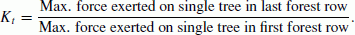((9))

This was dictated by the fact that no clear transmitted wave height could be determined in case of the development of the solitons train resulting from the fission process.

As indicated in Fig. 12, where K t is plotted against relative forest width B/L i,gen (L i,gen : the generated/measured wave length in front of the slope model, see Fig. 6), transmission of solitary wave through the mangrove forest is significantly influenced by the evolution modes which are governed primarily by the incident wave/water depth conditions. The highest transmission coefficient corresponds to non-breaking wave (i.e. evolution mode “EM1”) of height H i,nom = 0.04 m, propagating in water depth of h = 0.565 m over forest width B = 2.25 m. A much higher wave reduction is achieved in case of breaking wave conditions (i.e. evolution modes “EM2”–“EM3”) due to the additional source of energy losses caused by turbulent processes accompanying the breaking event. The lowest transmission coefficient (K t ~ 0.21) was observed for wave of height H i,nom = 0.08 m, generated at water depth h = 0.465 m and breaking in front of the forest (“EM3”) of width B = 3.0 m.

Generally, there is a trend of decreasing of wave transmission with the increasing relative forest width, which is particularly noticeable when comparing the transmission coefficients for the smallest (B = 0.75 m) and the largest forest widths (B = 3.0 m). For example, for water depth h = 0.515 m and incident wave height H i,nom = 0.16 m the transmission coefficient yields K t = 0.763 in case of forest width B = 0.75 m and it is reduced to K t = 0.488 in case of forest width B = 3.0 m. Such a high rate of wave attenuation in case of breaking waves results also from the fact that the measurements of the forces exerted on single tree models were always performed over the entire considered forest width (see exemplary the configuration of the force transducers for single tree models in Figs. 10 and 11). Thus, a much longer wave propagation distance was covered by the force measurements for the widest forest, so that more significant wave energy reduction took place as compared to the shortest forest. Additionally, transmission coefficient may vary even by a factor of 3 for a similar ratio of relative forest width B/L i,gen , depending on the evolution mode (as indicated by the lower and the upper envelope in Fig. 12).

The calculated values of the transmission coefficient are provided in Table A.1 in Appendix A for each of the performed tests.

The influence of the shore topography to the transmission coefficient has been investigated separately by means of water surface measurement analysis from the 1 m-wide flume (without the forest model) (Daenecke, 2010). For this purpose, the total energy of the solitary wave was calculated based on the following relationship: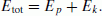((10))

The potential and kinetic energy can be calculated according to Longuet-Higgins and Fenton (1974) and Liu and Cheng (2001):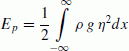((11))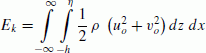((12))

where Etot is the total wave energy, E k the kinetic energy, E p the potential energy, u o the particle velocity in horizontal direction, v o the particle velocity in vertical direction, ρ the water density, g the gravity acceleration, and η the water surface elevation.

In this analysis, only the particle velocity in the direction of wave propagation (u o ) is considered and according to Munk (1949), linearization of mean particle velocity in horizontal direction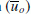can be determined as follows: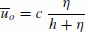((13))

where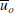is the mean particle velocity in horizontal direction, C the wave celerity, h the water depth, and η the water free surface elevation.

Based on the energy conservation law, the transmission coefficient is defined as the square root of the ratio of the transmitted and the incident wave energy: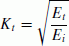((14))

where K t is the transmission coefficient, E i the incident wave energy and E t the transmitted wave energy.

Water surface elevation measured by wave gauges 6a and 7a is the input for the analysis (see Fig. 6(a)). By considering all forest widths, water depths h, and solitary wave heights H i,nom , the transmission coefficient K t is plotted in Fig. 13 as a function of relative forest width B/L i,gen (in the 1 m-wide flume without the forest). Since there is no forest model in the 1 m-wide flume, forest width B as shown in Fig. 13 is based on the forest width in the 2 m-wide flume in order to have comparable results.

From Fig. 13, it is clearly observed that K t based on the energy analysis is generally larger than K t based on the force measurements inside the forest model, particularly for B/L i,gen > 0.4 (see also Fig. 12). This indicates that the foreshore model may dissipate much of the wave energy, particularly for smaller B/L i,gen values and confirms field findings from Chatenoux and Peduzzi (2005) that the shore topography might reduce the impact of tsunami significantly. The role of the forest model in tsunami damping increases as relative forest width B/L i,gen increases (Fig. 14). The highest K t values for the upper and the lower envelopes of both results are almost similar because at these points, the waves are in non-breaking conditions (EM1) in which the equations used also valid solely for non-breaking wave conditions. More detailed results also obtained for regular and irregular wave trains which confirm the substantial contribution of the foreshore topography to the total wave damping performance (Husrin et al., 2011).

The transmission coefficients presented in this paper are comparable to the previously reported values from laboratory experiments, particularly for the range of K t values. Harada et al. (2000) reported K t values for porous media ranges from 1.0–0.5, while Kongko (2004) obtained the K t in the range between 0.95 and 0.65 for mangrove models made of a group of cylinders. Using wires-made mangrove models, Istiyanto et al. (2003) obtained a wider range of K t values (0.95–0.20). It should be noted here that those K t values were derived based on different parameterized tree models as well as different foreshore models. Moreover, their models also did not take into account the influence of breaking wave conditions. For breaking and non-breaking conditions, however, Augustin et al. (2008) reported K t values for a group of dowels subjected by irregular waves in the range of 0.99–0.65. Additionally, the highest transmission coefficient was determined for non-breaking wave conditions, which is in agreement with the results of the present study.

## 6. Conclusions

Two aspects of the damping performance of the mangrove forest have been investigated: (i) the development of the parameterization procedure to determine the tree model with an identical hydraulic resistance as its actual counterpart, which could be further used in the large scale model tests, (ii) the determination of the effect of the incident wave parameters, water depth conditions and forest width on wave reduction by the forest. The successful application of the parameterization procedure to a mature Rhizophora tree (the roots and the trunk according to the stiff structure assumption) revealed that the hydraulic resistance of the tree (drag coefficient C D ), tested under steady flow conditions, is influenced by submerged root volume ratio V m /V and tree frontal area A f . A new relationship between the drag coefficient based on effective length L e and Reynolds number R e is also provided.

The capability of the mangrove forest to damp tsunami was determined on the basis of large scale experiments with and without the forest model, which consisted of the selected parameterized tree models for different incident solitary wave conditions, water depths and forest widths. The rate of wave attenuation, analysed in terms of the forces exerted on single tree models, was found to be governed by the observed wave evolution modes, generally classified as non-breaking and breaking conditions. The highest wave energy reduction by forest model was achieved for breaking waves propagating over the widest forest (transmission coefficient K t = 0.2).

### List of Symbols

A f :::

frontal area of mangrove model [m2]

B::

width of mangrove forest model [m]

c::

wave celerity [m/s]

C D ::

drag coefficient [-]

d r ::

submergence depth of mangrove forest [m]

D t ::

cylinder diameter for the trunk model [m]

D c ::

cylinder diameter for the root model [m]

Etot::

total energy per unit crest width [J/m]

E k ::

kinetic energy per unit crest width [J/m]

E p ::

potential energy per unit crest width [J/m]

F::

measured force [N]

F D ::

measured drag force [N]

FD(par)::

measured force of parameterized mangrove model [N]

FD(real)::

measured force of real mangrove model [N]

Fmax::

maximum measured force on three model [N]

h::

water level [m]

H i,nom ::

nominal incident wave height [m]

H i,gen ::

generated incident wave height [m]

h tr ::

height of tree trunk [m]

K t ::

wave transmission coefficient [-]

LAI::

leave area index [-]

L::

wave length [m]

L e ::

effective length [m]

L i,nom ::

nominal incident wave length [m]

L i,gen ::

generated incident wave length [m]

P::

wave-induced pressure [kPa]

q::

relative flow discharge [m3/s/m2]

R e ::

Reynolds number [-]

u::

current flow velocity (depth-averaged velocity) [m/s]

u o ::

particle velocity in the direction of wave propagation [m/s]::

mean particle velocity in the direction of wave propagation [m/s]

v::

particle velocity perpendicular to the direction of wave propagation [m/s]

V::

control volume of water [m3]

V m ::

volume of submerged roots [m3]

x′::

horizontal distance of force transducers within the forest model [m]

δF D ::

deviation of measured force [%]

η::

water free surface elevation [m]

ν::

kinematic viscosity of water (ν = 1.004 × 10−6 m2/s for water temperature of 20°C)

ρ::

water density [kg/m3]

## References

1. Augustin, L. N., J. L. Irish, G. Balsmeier, and J. Kaihatu, Laboratory measurments of wave attenuation and wave setup by vegetation, Proceedings of the 31st International Conference on Coastal on Coastal Engineering ICCE, Hamburg, German, 324–330, 2008.

2. Brühl, M. and H. Oumeraci, Analysis of soliton fission over a submerged structure using Non-linear Fourier Transform, Proceedings of the 32nd International Conference on Coastal on Coastal Engineering, Shanghai, China, 12 pp, 2010.

3. Chatenoux, B. and P. Peduzzi, Analysis of the role of bathymetry and other environmental parameters in the impacts from the 2004 Indian Ocean Tsunami, UNEP/GRID-Europe, 25 pp., 2005.

4. Daenecke, K., Solitary wave attenuation anlysis by mangrrove forest using energy dissipation approach, Master thesis, Leichtweiss Institute for Hydraulic Engineering and Water Resources, TU Braunschweig, Germany, 121 pp., 2010.

5. Dahdouh-Guebas, F., L. P. Jayatissa, D. Di Nitto, J. O. Bosire, D. Lo Seen, and N. Koedam, How effective were mangroves as a defence against the recent tsunami?, Curr. Biol., 15(12), 443–447, 2005.

6. Environmental Justice Foundation (EJF), Mangroves: Nature’s defence against tsunamis—A report on the impact of mangrove loss and shrimp farm development on coastal defences, Environmental Justice Foundation (EJF) Report, London, UK, 30 pp., 2006.

7. Fritz, H. M., J. C. Borrero, C. E. Synolakis, and J. Yoo, 2004 Indian Ocean tsunami flow velocity measurements from survivor videos, Geophys. Res. Lett., 33(24), 5 pp., 2006.

8. Grant, P. F. and W. G. Nickling, Direct field measurement of wind drag on vegetation for application to windbreak design and modeling, Land Degradation and Development, 9, 57–66, 1998.

9. Harada, K. and F. Imamura, Experimental study on the resistance by mangrove under the unsteady flow, Proceedings of the 1st Congress of the Asian and Pacific Coastal Engineering, Dalian, 975–984, 2000.

10. Harada, K., H. Latief, and F Imamura, Study on the mangrove control forest to reduce tsunami impact, Proceedings of the 12th Congress of the IAHRAPD, Bangkok, Thailand, 10 pp., 2000.

11. Husrin, S. and H. Oumeraci, Parameterization of coastal forest vegetation and hydraulic resistance coefficients for tsunami modelling, Proceedings of the 4th Annual International Workshop and Expo on Sumatra Tsunami Disaster and Recovery, Banda Aceh, Indonesia, 78–86, 2009.

12. Husrin, S. and H. Oumeraci, Mangrove parameterization with stiff strucutre assumption A hydraulic model study, Progress Report, Leichtweiss Institute for Hydraulic Engineering and Water Resources, TU Braunschweig, Germany, 46 pp, 2010.

13. Husrin, S., A. Strusińska, A. Hoque, and H. Oumeraci, Storm wave attenuation and damping performance of mangrove forest, e-Proceedings of the Joint Convention Makassar, Exploring Eastern Indonesia, 16 pp, 2011.

14. Imai, K. and H. Matsutomi, Fluid force on vegetation due to the tsunami flow on sand spit, in Tsunamis: Case Studies and Recent Developments, edited by K. Satake, 343 pp, Springer, The Netherlands, 2005.

15. Istiyanto, D. C., K. S. Utomo, and Suranto, Pengaruh Rumpun Bakau Terhadap Perambatan Tsunami di Pantai, Proceeding of Reducing the Impact of Tsunami Seminar, BPPT JICA, Yogyakarta, Indonesia, 316– 326, 2003 (in Indonesian).

16. Kongko, W., Study on tsunami energy dissipation in mangrove forest, 43 pp., Master Thesis Report, Iwate University, Japan, 2004.

17. Liu, P. L.-F and Y. Cheng, A numerical study of the evolution of a solitary wave over a shelf, Phys. Fluids, 13(6), 1660–1667, 2001.

18. Longuet-Higgins, M. S. and J. D. Fenton, On the mass, momentum, energy and circulation of a solitary wave, Proc. R. Soc. Lond., A, 337, 1–13, 1974.

19. Mazda, Y., E. J. Wolanski, B. A. King, A. Sase, D. Ohtsuka, and M. Magi, Drag force due to vegetation in mangrove swamps, Mangroves and Salt Marshes, 1(3), 193–199, 1997.

20. Munk, W., The solitary wave theory and its application to surf problems, Ann. NY Acad. Sci., 51, 376–424, 1949.

21. Strusinska, A., Hydraulic Performance of an Impermeable Submerged Structure for Tsunami Damping, 228 pp, Ibidem-Verlag, Germany, 2011.

22. Struve, J., R. A. Falconer, and Y. Wu, Influence of model mangrove trees on the hydrodynamics in a flume, Estuar. Coast. Shelf Sci., 58,163–171, 2003.

23. Tanaka, N., Y. Sasaki, M. I. M. Mowjood, K. B. S. N. Jinadasa, and S. Homchuen, Coastal vegetation structures and their functions in tsunami protection: Experience of the recent Indian Ocean tsunami, Landsc. Ecol. Eng., 3, 33–45, 2007.

24. Yanagisawa, H., S. Koshimura, K. Goto, T. Miyagi, F Imamura, A. Ruangrassamee, and C. Tanavud, The reduction effects of mangrove forest on a tsunami based on field surveys at Pakarang Cape, Thailand and numerical analysis, Estuar. Coast. Self Sci., 81, 27–37, 2009.

## Acknowledgments

This study was performed in the framework of the project “Tsunami Attenuation Performance of Coastal Forests” (TAPFOR), a subproject of the project “Tracing Tsunami impacts on- and offshore in the Andaman Sea Region” (TRIAS), founded by the Deutsche Forschungsgemeinschaft (DFG) and the Office of the Research Council of Thailand (NRCT). The first author is also supported by the DFG within the Graduate College of TU Braunschweig (GRK 802) in “Risk Management of Natural & Civilization Hazards on Buildings & Infrastructure”. The authors would like to thank students H. Brodersen, D. Schubert and A. Syukri for their support in conducting of the experiments.

## Author information

Authors

### Corresponding author

Correspondence to Semeidi Husrin.

## Rights and permissions

Reprints and Permissions

Husrin, S., Strusińska, A. & Oumeraci, H. Experimental study on tsunami attenuation by mangrove forest. Earth Planet Sp 64, 15 (2012). https://doi.org/10.5047/eps.2011.11.008

• Revised:

• Accepted:

• Published:

### Key words

• Tsunami attenuation
• mangroves
• tree parameterization
• laboratory experiments